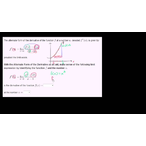# Class 11 Physics (India): Worked example: Derivative from limit expression

Need more information? Get more details on the site of the provider.### Description

Sal interprets a limit expression to find that it describes the derivative of f(x)=x³ at the point x=5.

## Class 11 Physics (India)

Let's learn, practice, and master topics of class 11 physics (NCERT) starting with kinematics and then moving to dynamics with Newton's laws of motion, work, energy, and power. Let's then use these as the foundation to learn about centre of mass, rotational motion, gravitation, solids, fluids, thermodynamics, and oscillations and waves.

## Derivative as a limit

There are two ways to define the derivative of function f at point x=a. The formal definition is the limit of [f(a+h)-f(x)]/h as h approaches 0, and the alternative definition is the limit of [f(x)-f(a)]/(x-a) …

There are no frequently asked questions yet. If you have any more questions or need help, contact our customer service.

Didn't find what you were looking for? See also: Physics, Teaching Skills, Biology, Mathematics, and Science.

Sal interprets a limit expression to find that it describes the derivative of f(x)=x³ at the point x=5.

## Class 11 Physics (India)

Let's learn, practice, and master topics of class 11 physics (NCERT) starting with kinematics and then moving to dynamics with Newton's laws of motion, work, energy, and power. Let's then use these as the foundation to learn about centre of mass, rotational motion, gravitation, solids, fluids, thermodynamics, and oscillations and waves.

## Derivative as a limit

There are two ways to define the derivative of function f at point x=a. The formal definition is the limit of [f(a+h)-f(x)]/h as h approaches 0, and the alternative definition is the limit of [f(x)-f(a)]/(x-a) as x approaches a. Make introduction with these two definitions.

## Topic: Science

Learn about all the sciences, from physics, chemistry and biology, to cosmology and astronomy, across hundreds of videos, articles and practice questions.

There are no reviews yet.
• View related products with reviews: Physics.